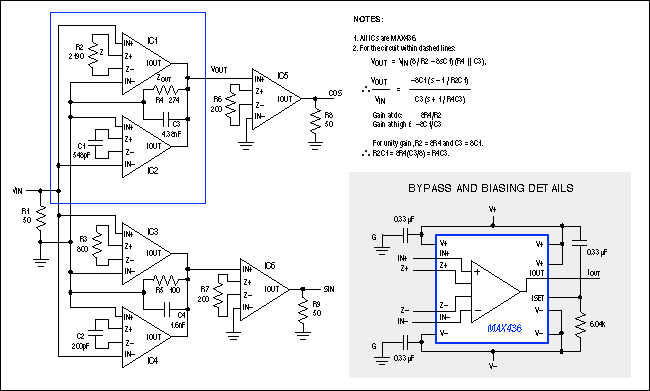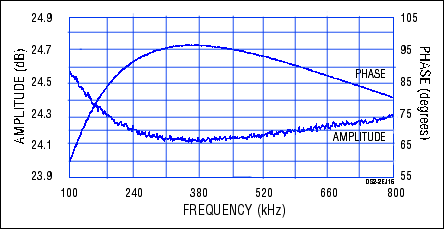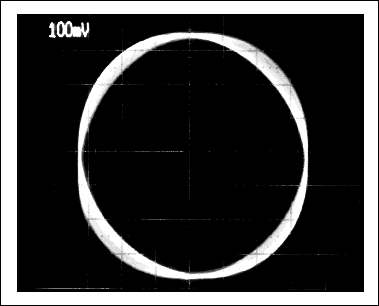# Allpass Network Shifts 90 Degrees over Wide Frequency Range

### Abstract

Allpass networks are widely used in communications and signal-processing applications. An example is the 90 degree phase-shift network used (with mixers) to produce a single-sideband signal. In Figure 1, the two allpass circuits have corner frequencies (determined by the output RC networks) that differ by a factor of 7.5. The result is an output phase difference that remains close to 90 degrees over a wide frequency range.

Unlike lowpass, bandpass, and other magnitude-shaping filters, allpass filters are able to shift the phase of a signal without affecting its amplitude. For a first-order allpass, the transfer function is as follows:As you sweep the variable "s" from zero (DC) to infinity, the sign of H(s) changes from plus to minus, indicating a change in phase from zero to 180°. You can implement this function with two wideband transconductance amplifiers (WTAs), as shown within the dotted lines of Figure 1.Figure 1. Two wideband transconductance amplifiers (dotted lines) produce an allpass network. Combining two such networks as shown produces two outputs with a constant 90° phase shift (vs. frequency) between them.

The WTA's transfer function is IOUT = 8VIN/Z, where "8" is an internal constant and Z is the gain-setting impedance. Most transconductance-amplifier applications require a resistive Z, but the WTA has an unusual capability that enables synthesis of the allpass function: It lets you connect an inductor, a capacitor, or any other impedance network for Z. VOUT = IOUTZOUT, so the transfer function for voltage amplification is VOUT/VIN = 8(ZOUT/Z). Unity gain demands Z = 8ZOUT, as shown.

The allpass circuit combines a resistive-Z WTA (IC1) with a capacitive-Z WTA (IC2). At low frequency, the circuit's output current is dominated by IC1, because C1's high impedance produces low IOUT from IC2. Rising frequency lowers this impedance, causing the current from IC2 to dominate at high frequencies. Moreover, IC2 inverts and IC1 does not, which provides the desired effects of noninverting unity gain at DC and inverting unity gain at high frequency.

Allpass networks are widely used in communications and signal-processing applications. An example is the 90° phase-shift network used (with mixers) to produce a single-sideband signal. In Figure 1, the two allpass circuits have corner frequencies (determined by the output RC networks) that differ by a factor of 7.5. The result is an output phase difference that remains close to 90° over a wide frequency range.

This allpass performance can be illustrated in two ways. The network response (Figure 2) shows 0.2dB amplitude variations and a phase difference of 90° ±7° from 180kHz to 740kHz, a 4:1 range. An oscilloscope's XY display offers another way to assess the deviations from 90°: Constant 90° produces a circle, and phase deviations cause a thickening of the trace, as shown in Figure 3. The photo represents an input-frequency sweep from 100kHz to 800kHz.Figure 2. This network response for Figure 1 shows a ±7° deviation in phase and a 0.2dB deviation in amplitude over the range 180kHz to 740kHz.Figure 3. The XY display of an oscilloscope gives another measure of performance for the Figure 1 circuit (a perfect circle of uniform thickness indicates a constant 90° phase shift).